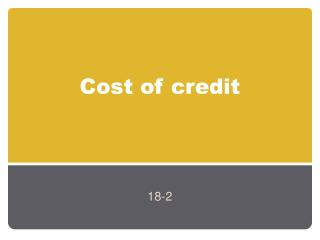DownloadDownload PresentationCost of credit

# Cost of credit

Télécharger la présentation## Cost of credit

- - - - - - - - - - - - - - - - - - - - - - - - - - - E N D - - - - - - - - - - - - - - - - - - - - - - - - - - -

1. ### Cost of credit

18-2
2. Finding interest Borrowing money has a cost Interest (I) is the cost of using someone else’s money. The amount of interest paid on a loan should be clearly understood. To determine the amount you need the following Principal (P) Amount of the loan Interest Rate (R) Percent of interest charged or earned. Time (T) Length of time for which interest will be charged. Usually in years or parts of years.
3. Simple interest Used on single payment loans Formula: Interest= Principal x Rate x Time I = P x R x T
4. Simple interest example Suppose you borrow \$100 (P) at 12% (I) for 1 year (T). I = P x R x T I= \$100 x 0.12 x 1 = \$12 In total you would pay \$112
5. Time in months Your loan may be for 1 month instead of a year. How do you calculate simple interest for less than a year. There are 12 months in a year so one month would be 1/12.
6. Time in months example If you borrow \$100 at 12% for one month I = P x R x T I= \$100 x 0.12 x 1/12 = \$1
7. Time in Days A loan may be for a certain number of days such as 30, 60, or 90. To make it easy think of a year as 360 days. If you borrow \$100 at 12% for 60 days I = P x R x T I= \$100 x 0.12 x 60/360
8. Maturity dates Is the date when the loan must be paid back If the loan is stated in months the date of maturity is the same day of the month as when the loan was made. i.e. a one-month loan made on Jan 15 will be due on Feb 15. When the time is in days you must count the exact number of days to find when it is due. See page 458 for an example of finding a 90 day loan.
9. Installment Interest Is a loan that you make in several payments instead of 1 large payment. Usually offered by banks, credit unions, and consumer finance companies. The bank gives you a schedule of payments You must sign a promissory note. You borrow \$100 and sign a note for \$110 \$10/\$100 = .10 or 10% interest. 110/12= \$9.17 this is your monthly payment
10. Decreasing loan payments Some loan installments interest is calculated at the end of the month on the unpaid balance. Each month you would calculate I= P x R x T The principal would be the only thing different each month. See example on pg 18-2
11. Annual Percentage Rate (APR) By law all credit agreements must state the percentage cost of credit on a yearly basis. In addition to interest the APR includes other service charges such as service fees. Uncollectable accounts or bad debt may be passed on to other borrowers. Lenders may add an amount to cover the cost of credit insurance This covers the amount owed if the borrower dies.
12. Total Dollar Charges To make you aware of the total cost of credit, federal law requires that the lender must tell you the finance charge The finance charge is the total dollar cost of credit including interest and all other charges. Either your contract or your charge account statement must state this finance charge.
13. Compare Credit costs If you need to borrow money or buy on credit, be sure to compare the total cost of credit among lenders. Check APR If you make a purchase with a credit card make sure you use the one with the lowest APR When getting a loan make sure you compare APR, Total cost and monthly payments. Always remember when using credit you are spending with future income.